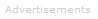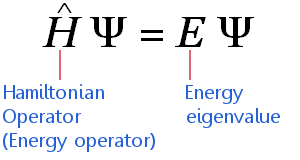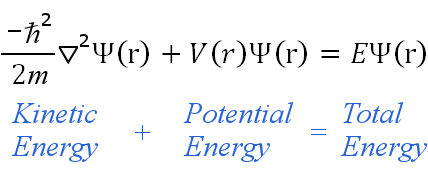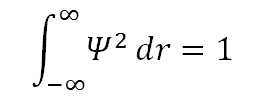# Definition of the Schrödinger Equation

The Schrödinger equation, sometimes called the Schrödinger wave equation, is a partial differential equation. It uses the concept of energy conservation (Kinetic Energy + Potential Energy = Total Energy) to obtain information about the behavior of an electron bound to a nucleus. It does this by allowing an electron's wave function, Ψ, to be calculated.Solving the Schrödinger equation gives us Ψ and Ψ2. With these we get the quantum numbers and the shapes and orientations of orbitals that characterize electrons in an atom or molecule.

The Schrödinger equation gives exact solutions only for nuclei with one electron: H, He+, Li2+, Be3+, B4+, C5+, etc. In mathematical language, we say that analytic solutions for Ψ are possible only for one-electron systems. One-electron systems are often described as hydrogenic - meaning "like hydrogen."

For all other atoms, ions, and molecules, no analytic solutions for Ψ are possible; approximation methods of calculation, such as the orbital approximation and variation theorem, are then utilized.

There is a time-dependent Schrödinger equation and a time-independent Schrödinger equation.

The time-independent equation considers the electron's quantum state to be unchanging, hence it considers the electron as a standing wave. The time-independent equation allows electron densities (i.e. the sizes and shapes of atomic and molecular orbitals) to be found using Ψ2, the square of the wave function.

The p orbitals below are examples of Ψ2:

pxpypz## The Time-Independent Schrödinger Equation

The time-independent Schrödinger equation can be expressed in highly compressed mathematical shorthand as:The equation applies to electrons traveling at non-relativistic speeds. (This means it requires adjustments before it can be applied high mass elements.) The equation says:

The sum of the Wave Function's Kinetic Energy and Potential Energy = The Wave Function's Total Energy

The time-independent equation can be written in any suitable coordinate system, such as Cartesian coordinates (x,y,z). For hydrogenic atoms, spherical polar coordinates are more suitable, hence:is the reduced Planck constant,
m is the electron mass,is the Laplacian operator,
Ψ is the wave function,
V is the potential energy,
E is the energy eigenvalue,
(r) denotes the quantities are functions of spherical polar coordinates (r, θ, φ)

### Constraints Result in Quantization

The equation is solved to find Ψ. Constraints placed on solving the equation produce quantization - i.e. the solutions found for Ψ are restricted to certain values and all other values are forbidden.

These constraints are:

Ψ and its first partial derivative must be continuous.

Ψ must be normalizable: this means there is a 100 percent probability of the electron being somewhere in the universe. For a real valued Ψ, normalization requires that:As the distance from the nucleus increases, the electron becomes detached and is no longer bound. i.e. as r → ∞, H → H+ + e-.

### The Wave Function Produces Quantum Numbers

The electron's wavefunction exists in three dimensions, therefore solutions of the Schrödinger equation have three parts. These are obtained explicitly by a method of solving partial differential equations called separating the variables. Doing this, we get:

Ψ(r, θ, φ) = R(r) P(θ) F(φ)

It turns out that solutions for Ψ are only possible when:

In R(r), a constant, call it n, has values 1, 2, 3, 4,....

In P(θ), a constant, call it l, has values 0, 1, 2, 3,...(n-1)

In F(φ), a constant, call it ml, has values -l, (l+1),...0..., (l+1), l

And so from the wave function Ψ the Schrödinger equation has delivered the three quantum numbers that characterize electron behavior in an atom.

n: the principal quantum number

l: the orbital angular momentum quantum number

ml: the magnetic quantum number

### The Wave Function Produces Orbital Shapes and Sizes

Ψ2, the probability density, defines the shapes and sizes of electron orbitals.Search the Dictionary for More Terms